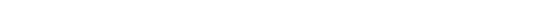NEWS NLREG has been selected as the "Editor"s Pick" by SoftSeek.NLREG is in use at hundreds of universities, laboratories, and government agencies around the world (over 20 countries). For a list of a few organizations using NLREG click here. If you have categorical variables, you may want to use a Decision Tree to model your data. Check out the DTREG Decision Tree Builder. You also should check out the News Rover program that automatically scans Usenet newsgroups, downloads messages of interest to you, decodes binary file attachments, reconstructs files split across multiple messages, and eliminates spam and duplicate files.## Piecewise Function With Two Segments

Fit a function consisting of two linear segments that bend at X=5. When X is less than 5, the slope of the function is B1. When X is greater than or equal to 5, the slope is B2. B0 is the Y value of the function at X=5 (i.e., at the pivot point). The if statement is used to control which function model is used depending on whether the value of the dependent variable is greater than or less than the pivot point.

```Title "Piecewise linear function";
Variables X,Y;
Parameter B0;
Parameter B1;
Parameter B2;
Constant Pivot=5;		// X value at which bend occurs
if (x < Pivot) {
Function Y = B0+B1*(X-Pivot);
} else {
Function Y = B0+B2*(X-Pivot);
}
Plot residual;
Rplot grid;
Data;
[ data goes here ]
```